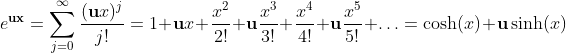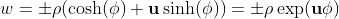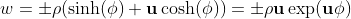Wacker Art Geometric Algebra and MatricesBild: "Passat Travemünde"

### Attention

This page has been tested and developed with the Mozilla browser. This page requires JavaScript in your browser. Without JavaScript or JavaScript beeing disabled, the display of this side is incomplete.

## Prolog

Die rote oder die blaue Pille?

### References

This page is based on the concepts developed on my geometric algebra page and on my linear algebra page.

## Matrix Representation of the Canonic Base Elements of the Geometric Algebra 𝔾2

A vector v ∈ ℝ2 can be written in the following form, using the unit vectors e1, e2:

v = v1e1 + v2e1

From the base vectors e1, e2 the canonic elements of the geometric algebra 𝔾2 can be derived.

Scalar: I = I(0), Vectors: e1, e2, Pseudoscalar(element with highest grade): I(2)=e1e2=e1e2.

### Algebraic Relations of the Canonic Base Elements in the Geometric Algebra 𝔾2

(e1e2)2 = (e1e2)2 = -I;

e12 = I;

e22 = I;

(e1e2)e2 =  e1;

e2(e1e2) = -e1;

e1(e1e2) =  e2;

(e1e2)e1 = -e2;

-e1e2 = -(e1e2) = e2e1 = e2e1;

(e2e1)2 = (-e1e2)2 = -I

### Matrix Form of the Canonic Elements of 𝔾2

The canonic elements of the geometric algebra 𝔾2 can be written in matrix form. These matrices have the same algebraic properties as the canonic elements of 𝔾2.

These four matrices form a group.

The three matrices are the same as the three matrices for reflection and 90° rotation. Visit the rotation matrix page for details.

### Algebraic Relations between 𝔾2 Base Elements and the Matrix Representation of these Elements

(e1e2)2 = (e1e2)2 = -I;

e12 = I;

e22 = I;

(e1e2)e2 =  e1;

e2(e1e2) = -e1;

e1(e1e2) =  e2;

(e1e2)e1 = -e2;

-e1e2 = -(e1e2) = e2e1 = e2e1;

## Complex Numbers as Matrices

A complex unit called i is the solution of the expression:

x2 + 1 = 0; x2 = -1; x = ±-1 = ±i;

and has the property:

i)2 = -1

### The Product of two Complex Numbers as Matrix Product

Using the matrix representation of two complex numbers z1, z2 will give the following product:

### Polar Form of a Complex Number

The exponential presentation of a complex number z looks as follows:

z = eriθ = ereiθ = er(cos(θ) + isin(θ)) = ercos(θ) + iersin(θ)

Expressing this equation in matrix form will give:

## Hyperbolic Numbers in Matrix Form

Hyperbolic unit called u is the solution of the expression:

x2 - 1 = 0

and has the following properties:

u2 = 1 and u ≠ ±1

A hyperbolic number w can be expressed as matrix.

It has the conjugate w that has the following matrix form:

Which is equivalent to:

ww = (x+uy)(x-uy) = x2-y2.

### Hyperbolic Polar Form

Exponential function with hyperbolic unitHypebolic numbers can easiely expressed in hyperbolic polar form.

For quadrants I and III we have:For quadrants II and IV we have:### Hyperbolic Units in Matrix Form

uu = uu = u(-u) = (-u)u = -I

### Polar Form of a Hyperbolic Number

w = eruθ = ereuθ = er(cosh(θ) + usinh(θ)) = ercosh(θ) + uersinh(θ);

Expressing this equation in matrix form will give:

## Chromogeometry - Complex Numbers in Matrix Form

Chromogeometry is a generalisation of the matrix representation of complex numbers. Also known as split quaternions.

When you take the building matrices for the matrix representation of the geometric algebra 𝔾2:

it is possible to construct three different subalgebras named blue, red, green:

These matrices build commutative subalgebras of the 2x2 matrices.

They have the properties:

 i2 = -I u2 = I h2 = I ui = h, iu = -h ih = u, hi = -u uh = i, hu = -i

The three Matrices blue red and green can be interpretated as two reflections in the blue and red case and as a rotation in the green case.

### Dihedron Algebra

This is a quadratic invers algebra over a field 𝔽 an algebra with a multicative quadratic form that controls the inverses.

D𝔻

### Relation between a Determinant and a Quadratic Form

Only in the case of the 2x2 matrices there is a special relation between the trace of a matrix A and a quadratic form Q(A).

det(A) = ad-bc = Q(A);   (In 2-dimensions the determinant is equal to a quadratic form)

A1, A2 ∈ 𝔻;

det(A1A2) = det(A1)det(A2);

det(A) = Q(A) =>

Q(A1A2) = Q(A1)Q(A2);

### Polarisation Identity

w, v ∈ 𝔻;

Q(w+v) = (w+v)·(w+v) = w·w + v·v + 2w·v;

2w·v = Q(w+v) - Q(w) - Q(v);

In the two dimensional case the quadratic form Q(v) = det(v) (only in 2 dimensions):

2w·v = det(w+v) - det(w) - det(v)

### Scalar Product and the Trace

Take the sum of two dihedrons

Q(r1+r2) = det(r1+r2) = (a1+a2)(d1+d2) - (b1+b2)(c1+c2);

Q(r1) = det(r1) = a1d1-b1c1

Q(r2) = det(r2) = a2d2-b2c2

Calculating the scalar product by the use of the polarisation identity:

2r1·r2 = det(r1+r2) - det(r1) - det(r2) = a1d2 + a2d1 -b1c2 - b2c1;

Calculating the product of a Dihedron and the Adjoint of a Dihedron:

Calculating the trace of the product and compare it with the result of the scalar product gives:

 2r1·r2 = trace((r1)(r2)adj) = a1d2 + a2d1 - b1c2 - b2c1;

The result is also known as the scalar dot product.

The adjoint radj of a 2x2 matrix r is defined as follows:

### Dihedron Group

Set of the Dihedron Group: 𝔾: {±I, ±i, ±u, ±h}

Product of the Dihedron Group

## Idempotent Base

The standard base for hyperbolic numbers w is {1,u}. An alternative to this base is the idempotent base of the hyperbolic numbers w is the pair {u+, u-}.

Now we have two ways to represent a hyperbolic number:

The idempotent base elements are defined in the relation to the standard base elements as follows:

The new indempotent base elements {u+,u-} have the idempotent and the annihilation properties.

### Idempotent Property

The elements u+ and u- are idempotent because (u+)2 = u+ and (u-)2 = u-.

### Annihilating Property

The elements u+ and u- are mutually annihilating because u+u- = 0.

### Spectral Decomposition of Hyperbolic Numbers

The decomposition of w = w+u+ + w-u+ is the spectral decomposition of w = x + uy. Where the spectral weight elements w+ and w- are defined as follows:

The the weight elements x and y of standard base {1,u} of the hyperbolic numbers can be calculated from the weight elements w+, w- of the idempotent base {u+,u-}.

### Spectral Decomposition

With the following spectral components:

### Product

The product of the spectral weight element w+ and w- is:

### Applications

The product of two hyperbolic numbers is:

vw = (v+u+ + v-u-)(w+u+ + w-u-) = (v+w+)u+ + (v-w-)u-

The binomial theorem gives a very simple form with the idempotent basis. Hence the powers of hyperbolic numbers can be easy computed.

(w+u+ + w-u1)k = (w+)k(u+)k + (w-)k(u-)k = (w+)ku+ + (w-)ku-

## Pauli Matrices

The Pauli matrices contain the complex element i with the property i2 = -1.

### Properties of the Pauli Matrices

σ0 = I;

0)2 = (σ1)2 = (σ2)2 = (σ3)2 = I;

12)2 = (σ23)2 = (σ31)2 = -I;

σ1σ2σ3 = iI = I(2);

123)2 = (I(2))2 = -I

σ1σ2 = iσ3;

σ2σ3 = iσ1;

σ3σ1 = iσ2;

### Matrix Calculations

Preforming the matrix calculations:

### Pauli Matrices and Quaternions

The following relationship between the Pauli matrices and the quaternions exists:

i = iσ1; j = -iσ2; k = iσ3;

With these relations it is possible to define the quaternions as two by two matrices with complex elements:

### Pauli Matrices and the Geometric Algebra 𝔾3

The following relation between the Pauli matrices and the Geometric Algebra 𝔾3 exists:

eiej = δij + I(3)εijkek σiσj = δij + iεijkσk

Pauli matrices as 4x4 matrices can be found here:

## Matrix Representation of the Canonic Base Elements of the Geometric Algebra 𝔾3

More information on the geometric algebra 𝔾3 can be found on my geometric algebra page. The geometric algebra 𝔾3 has a strong relation to quaternions.

A vector v ∈ ℝ3 can be written in the following form, using the unit vectors e1, e2, e3 :

v = v1e1 + v2e1 + v3e3

Using 4×4 matrices to define I, e1, e2, e3 and the unit psuedoscalar e123 = I(3).

### Relation to Quaternions

The bivectors -e12, -e23, -e31 are equivalent to the quaternions. These bivectors have the same algebraic properties as the quaternions.

Using the unit pseudo scalar I(3) as imaginary unit we get the following relations to the quaternions:

i = -I(3)e1;   j = -I(3)e2;   k = -I(3)e3;

## Geometric Numbers

Geometric numbers comprise the real numbers and two new elements a,b with special properties. The new geometric numbers a,b also called g-numbers have the following properties:

(i)  a2=0=b2 The new g-numbers are called nilpotent or null vectors.

(ii) 2ab =ab+ba=1

#### The g-Numbers Expressed as Matrices

The canonic elements a and b expressed as matrices:

Squaring of the canonic matrices will result in the zero matrix:

a2 = 0;

b2 = 0;

Building the product a and b and the product b and a.

ab

ba

The products ab and ba expressed as matrices:

ab + ba = 1

ab - ba = -h

aba=(ab)a=(1-ba)a=a-ba2=a

bab=(ba)b=(1-ab)b=b-ab2=b

(ab)2=(1-ba)ab=ab+ba2b=ab

(ba)2=(1-ab)ba=ba+ab2a=ba

(ab)(ba) = 0 = (ba)(ab)

From the above properties the multiplication table for basic g-numbers a, b, ab, ba can be dereived.

 a b ab ba a 0 ab 0 a b ba 0 b 0 ab a 0 ab 0 ba 0 b 0 ba

The basic g-numbers can be written in a matrix form:

We have the following matrices for a, b, ab and ba:

The general form of a g-matrix is as follows:

A geometric number g can know be expressed as follows.

### Conjugation and Inversion

Each g-number can be seen as the sum of two parts g = go + ge the odd part go and the even part ge.

The odd part of a g-number looks as follows:

The even part of a g-number looks as follows:

### The reverse of a g-number

With (ab) = ba the reverse operation for the odd and the even part are defined as follows:

go = go

ge = g11ab + g22ba

The reverse of a g-number has the following property:

g = (go + ge) = go + ge = go + ge

Hence we have for g

### The inverse of a g-number

The invers of a g-numger is named g- and has the following properties:

g-o = -g12b - g21a; for the odd part.

g-e = ge; for the even part.

The sum of the even and the odd part will give g-

g- = (go + ge)- = g-o + g-e = -go + ge

Hence we have for g-

### Mixed conjugation

The combination of the operations of reversion and inversion of a g-number will give the mixed conjugation g*.

g* = (g)- = (go + ge)- = -go + ge

### Geometrie of a and b

Construction of the geometric algebra 𝔾2 with the nilpotent 2x2 matrices a and b.

The relations between these matrices can be found by the matrix representation of the geometric algebra 𝔾2

## Determinants and the Outer Product

### Outer Product of two Vectors and the Determinant

Bulding the outer product of two vectors u and v gives:

uv = (u1e1 + u2e2) ∧ (v1e1 + v2e2) = (u1v2 - u2v1)(e1e2);

The first term on the right side (u1v2 - u2v1) is the value of the determinant:

### Outer Product of three Vectors and the Determinant

Bulding the outer product of three vectors u, v and w gives:

uvw = (u1e1 + u2e2 + u3e3) ∧ (v1e1 + v2e2 + v3e3) ∧ (w1e1 + w2e2 + w3e3) = (u1v2w3 + v1w2u3 + w1u2v3 - w1v2u3 - v1u2w3 - u1w2v3) (e1e2e3);

The first term on the right side (u1v2w3 + v1w2u3 + w1u2v3 - w1v2u3 - v1u2w3 - u1w2v3) is the value of the determinant.

### Outer Product of n-Vectors and the Determinant

The outer product of n-vectors is the derminant of the matrix with the n vectors as column vectors multiplied with the unit pseudoscalar I(n)

Picture: "Old Ship Flensburg"

The next page is about hypercomplex numbers.5. März 2021 Version 2.0
Copyright: Hermann Wacker Uhlandstraße 10 D-85386 Eching bei Freising Germany Haftungsausschluß